Next: Small-Signal AC Model Up: The Junction Field Effect Previous: The Junction Field Effect

## Principles of Operation

Figure 5.15 shows an N-channel JFET with DC bias voltage applied. Just as for a simple diode, the depletion region grows as the reverse bias across the PN junction is increased, thereby constricting the cross section of the conducting N-channel material and increasing the resistance of the channel. The major current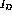in the channel is caused by the applied voltage between the drain and source,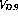, and is controlled by the applied voltage between the gate and source,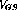.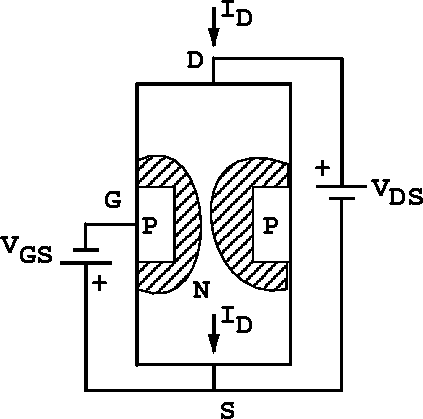Figure 5.15:  An N-channel JFET with DC bias voltages applied.

The JFET has two distinct modes of operation: the variable-resistance mode, and the pinch-off mode. In the variable-resistance mode the JFET behaves like a resistor whose value is controlled by. In the pinch-off mode, the channel has been heavily constricted with most of the drain-source voltage drop occuring along the narrow and therefore high-resistance part of the channel near the depletion regions.

The characteristic curves of a typical JFET are shown in figure 5.16. At small values of(in the range of a few tenths of a volt), the curves of constantshow a linear relationship betweenand. This is the variable-resistance region of the graph. Asincreases, each of the curves of constantenters a region of nearly constant. This is the pinch-off region, where the JFET can be used as a linear voltage and current amplifier. At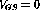the current through the JFET reaches a maximum known as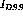, the current from Drain to Source with the gate Shorted to the source. Ifgoes positive for this N-channel JFET, the PN junction becomes conducting and the JFET becomes just a forward-biased diode.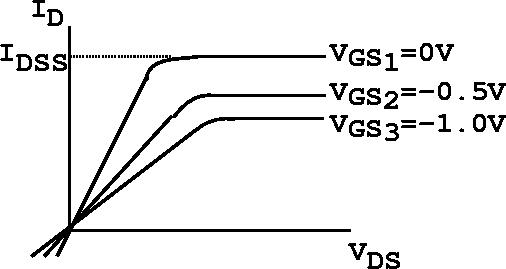Figure 5.16:  Characteristic curves of a typical N-channel JFET.Next: Small-Signal AC Model Up: The Junction Field Effect Previous: The Junction Field Effect

Doug Gingrich
Tue Jul 13 16:55:15 EDT 1999